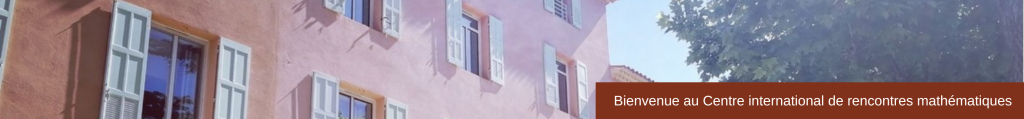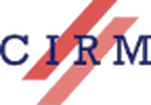RESEARCH SCHOOL / ECOLE DE RECHERCHE

Modeling and Control of Open Quantum Systems
Modélisation et contrôle des systèmes quantiques ouverts

16 – 20 April 2018

 Scientific Committee Comité scientifique Jean-Michel Coron (Université Pierre et Marie Curie)Michel Devoret (Yale University)​Lorenza Viola (Dartmouth College) Organizing CommitteeComité d’organisation Denis Bernard (LPT-ENS)John Gough (Aberystwyth University)Pierre Rouchon (Mines-ParisTech)
 This research school consists of a series of graduate-level courses presenting the mathematical models underlying and applicable to current experiments involving measurement and control of individual quantum systems. It addresses the following items that are crucial to developing a working understanding of open quantum system dynamics: spin/spring systems with dispersive/resonant coupling; quantum stochastic master equations, quantum trajectories and filtering; quantum Langevin equation and input/output theory; composite systems, entanglement, measurement, decoherence and dissipation. With respect to the standard university lectures, the choice of topics will aim at bridging the gap between mathematicians and physicists, presenting the requisite concepts and mathematical models that go beyond what is currently taught in general quantum physics courses, but staying closer to the experimental practices that are now emerging in quantum technologies. With this perspective in mind, this one-week school is based on  three 6-hour courses presenting the theoretical  modeling and control concepts  relevant to current  experiments. These courses are completed by three  3-hour lectures on two  kinds of experimental settings: cavity quantum electro-dynamical systems and superconducting circuits. Le trimestre IHP intitulé “Mesure et contrôle de systèmes quantiques: théorie et expériences” commence par cette école d’une semaine sur la modélisation et le contrôle des systèmes quantiques ouverts. Elle propose des cours et des conférences de niveau master sur les méthodes mathématiques et les modèles décrivant les expériences actuelles en mesure et contrôle quantique. Les cours présentent les éléments clés pour représenter et analyser la dynamique des systèmes quantiques ouverts: les modèles spin/ressort avec interaction dispersive ou résonante; les équations maîtresses stochastiques, les trajectoires quantiques et le filtrage; les équations quantiques de Langevin et la théorie entrée/sortie; les systèmes composites, l’intrication, la mesure, la décohérence et la dissipation. Les éléments choisis ont pour but de rassembler physiciens et mathématiciens. D’une part, ces éléments prolongent les méthodes mathématiques usuellement enseignées dans les cours de mécanique quantique. D’autre part, ils restent proches des problématiques expérimentales en ingénierie quantique. Cette semaine repose sur trois cours de six heures qui présentent les éléments théoriques en modélisation et contrôle, éléments adaptés aux expériences actuelles. Ces trois cours sont complétés par  trois conférences de trois heures sur deux types de  configurations expérimentales: l’électro-dynamique quantique en cavité et les circuits quantique supra-conducteurs. ​
Courses
Usual notations and some useful formulae for spin/spring composite quantum systems can be downloaded here.
Peter Zoller, Institute for Theoretical Physics, University of Innsbruck, and Institute for Quantum Optics and Quantum Information, Austrian Academy of Sciences, Innsbruck, Austria.

Introduction to Quantum Optics

Quantum optical systems provides one of the best physical settings to engineer quantum many-body systems of atoms and photons, which can be controlled and measured on the level of single quanta. In this course we will provide an introduction to quantum optics from the perspective of control and measurement, and in light of possible applications including quantum computing and quantum communication.
The first part of the course will introduce the basic quantum optical systems and concepts as ‘closed’ (i.e. isolated) quantum systems. We start with laser driven two-level atoms, the Jaynes-Cummings model of Cavity Quantum Electrodynamics, and illustrate with the example of trapped ions control of the quantum motion of atoms via laser light. This will lead us to the model system of an ion trap quantum computer where we employ control ideas to design quantum gates.
In the second part of the course we will consider open quantum optical systems. Here the system of interest is coupled to a bosonic bath or environment (e.g. vacuum modes of the radiation field), providing damping and decoherence. We will develop the theory for the example of a radiatively damped two-level atom, and derive the corresponding master equation, and discuss its solution and physical interpretation. On a more advanced level, and as link to the mathematical literature, we establish briefly the connection to topics like continuous measurement theory (of photon counting), the Quantum Stochastic Schrödinger Equation, and quantum trajectories (here as as time evolution of a radiatively damped atom conditional to observing a given photon count trajectory). As an example of the application of the formalism we discuss quantum state transfer in a quantum optical network.

Denis Bernard, Laboratoire de physique théorique, Ecole Normale Supérieure, Paris, France.

Statistical Aspects of Quantum State Monitoring for (and by) Amateurs

Upon monitoring a quantum system, one extracts recursively random output information and back-acts randomly on the system. These lectures are devoted to an introductory description of statistical aspects of these processes, which are instrumental in quantum state manipulation and control. We shall start with a description of recursive discrete indirect measurements, as exemplified by cavity QED experiments, including non-demolition measurement and their generalizations, and their connection with discrete quantum stochastic processes. We shall then study the formulation of continuous-in-time quantum monitoring via the so-called quantum trajectories and describe their basic properties and consequences. Illustrative examples will deal with simple quantum systems as well as with some many-body extended systems. Besides providing a framework for dealing with quantum state monitoring, these lectures aim at illustrating how the use of techniques from probability theory and stochastic processes helps deciphering and understanding physical consequences and applications of quantum state monitoring.

John Gough, Institute of Mathematics & Physics, Aberystwyth University, UK

Quantum Markovian Models, Input-Outputs, & Feedback Networks

The class of (continuous-time) Markovian models have merged as a natural one to treat quantum optics, cavity QED and several other key physical systems. From a conceptual point of view, they provide a framework for describing a quantum system driven by quantum inputs, and provide a clear relation to the quantum output processes – this is built on the quantum stochastic calculus, which we will also set out in the lectures, and allows us to introduce several methods from classical control theory naturally into the quantum domain. We will derive the general from of the theory – sometimes known as SLH models – and motivate this through key physical examples. Finally, we will show how networks of such components may be modelled and look at some recent proposals for quantum devices that are effectively networks of such quantum Markovian components: we will outline how these may enable future quantum technologies.

Lectures
Igor Dotsenko, Laboratory of Kastler and Brossel, Ecole Normale Supérieure and Collège de France, Paris, France.

Quantum trajectories and feedback in a photon box

Our photon box, i.e. a microwave cavity made of two very good mirrors, is capable of storing quanta of light for very long time. Individual, highly excited atoms, passing through the box one by one, serve us to manipulate and measure the photons’ quantum state in real time. This ultimate tool has been used in a wide range of quantum physics experiments. The aim of this lecture is to present the concept and realisation of several particular experimental protocols based on repetitive quantum measurements of trapped light followed by its quantum control.
I will first present you all relevant components of our experimental setup from both the experimental and the theoretical point of view. Atoms and photons, being our main quantum sub-systems, can be independently manipulated. Two basic interaction regimes between them, i.e. resonant for the energy exchange and dispersive for the quantum non-demolition (QND) measurement, will be then discussed. Finally, I will show you how we realise complete QND photon counting in the box, unveiling us, for instance, the wave function collapse and the quantum jumps of light.
In the second part of my lecture we will learn how to use the real-time QND information on the photons state in order to control its evolution. First, you will see the realisation of the quantum feedback algorithm stabilizing quantum states with fixed number of photons in the box. Here, ‘dispersive’ atoms are used to extract photon-number information and ‘resonant’ atoms to correct for quantum jumps if demanded by the real-time controller in a feedback loop. The second experiment to be discussed is the adaptive measurement of light where the actual intermediate knowledge on the field’s state is used to better choose the setting of the next single-atom measurement in order to speed up the state collapse, i.e. the preparation of photon-number states.

​(slides)

Benjamin Huard, Laboratoire de Physique, Ecole Normale Supérieure de Lyon, France

Quantum trajectories and feedback in circuit-QED

This lecture will provide an introduction to the field of superconducting circuits for quantum information processing. The analogy between circuit-QED (superconducting qubits in cavities) and cavity-QED (atoms in cavities) will be described. We will introduce continuous measurements by modeling homodyne and heterodyne measurements of a propagating field. Then, we will apply this model to two types of measurements: dispersive measurement and fluorescence measurement of a superconducting qubit. The corresponding stochastic master equation will be derived and we will show an experiment where it is used to observe quantum trajectories of a qubit in presence of one of both measurement schemes.
We will then discuss quantum feedback with superconducting circuits. First, we will talk about measurement based feedback on either the dispersive or the fluorescence measurement channels. We will see how Markovian feedback can help us stabilize permanently an arbitrary quantum state or quantum trajectory for a superconducting qubit. Then, we will show examples of autonomous feedback where an arbitrary state of a qubit can also be stabilized. If time allows, we will extend the discussions to more complex systems such as a pair of distant qubits where Bell states can be stabilized or harmonic oscillators where Schrödinger cat states can be stabilized.

(slides)

Pierre Rouchon, Mines-ParisTech, PSL Research University and Inria, Paris, France.

Quantum electrical circuits with capacitors, inductors and transmission lines

This lecture relies on the physical experiments and the mathematical methods presented in the 6-hour and 3-hour courses. It starts with the classical Euler-Lagrange and Hamiltonian formulation of typical electrical circuits made of linear capacitors/inductors and Josephson junction (nonlinear inductors) coherently driven and measured via semi-infinite transmission lines. The quantization of such circuits based on the correspondence principle is then detailed. Derivations of Langevin equations, SLH models and the stochastic master equations associated to homodyne/heterodyne detections of the output fields are finally addressed with a discussion of the involved approximations.